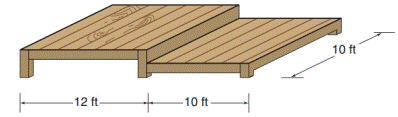Chapter 8.1, Problem 28EElementary Geometry For College St...

7th Edition
Alexander + 2 others
ISBN: 9781337614085

Solutions

Chapter
SectionElementary Geometry For College St...

7th Edition
Alexander + 2 others
ISBN: 9781337614085
Textbook Problem

Gary and Carolyn plan to build the deck shown.a) Find the total floor space (area) of the deck.b) Find the approximate cost of building the deck if the estimated cost is $6.40 per ft 2 .To determine a) To find: The total floor space (area) of the deck. Explanation Converting theoretical statement into mathematical statement, identifying the figure and the calculation is followed by suitable geometric formulae. Calculation: Given, Length of rectangular deck floor space l=(12+10)=22 ft Width of the rectangular deck floor space w=10 ft Total area of the rectangular deck To determine b) To find: The approximate cost of building the deck if the estimated cost is$6.40 per ft2.

Still sussing out bartleby?

Check out a sample textbook solution.

See a sample solution

The Solution to Your Study Problems

Bartleby provides explanations to thousands of textbook problems written by our experts, many with advanced degrees!

Get Started

In Exercises 1 and 2, simplify the expression. 5(2)34

Calculus: An Applied Approach (MindTap Course List)

Simplify: 1216

Elementary Technical Mathematics

Evaluate the expression sin Exercises 116. (23)2

Finite Mathematics and Applied Calculus (MindTap Course List)

For

Study Guide for Stewart's Multivariable Calculus, 8th